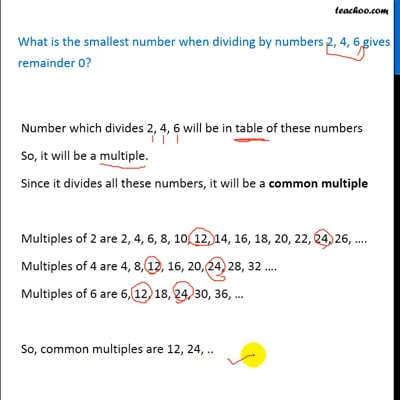Smallest square number divisible by numbers

Chapter 6 Class 8 Squares and Square Roots
Concept wise

Number which divides 2, 4, 6 will be in table of these numbers

So, it will be a multiple.

Since it divides all these numbers, it will be a common multiple

Multiples of 2 are 2, 4, 6, 8, 10, 12, 14, 16, 18, 20, 22, 24, 26, ….

Multiples of 4 are 4, 8, 12, 16, 20, 24, 28, 32 ….

Multiples of 6 are 6, 12, 18, 24, 30, 36, …

So, common multiples are 12, 24, ..

Here, we need to find the smallest number

So, Smallest number = 12

And this is the number which when divided by 2, 4, 6 gives remainder 0

And it is also the LCM – Least Common multiple

So, LCM = Number which gives remainder 0 when dividing by 2, 4, 6This video is only available for Teachoo black users

Maths Crash Course - Live lectures + all videos + Real time Doubt solving!

### Transcript

What is the smallest number when dividing by numbers 2, 4, 6 gives remainder 0? Number which divides 2, 4, 6 will be in table of these numbers So, it will be a multiple. Since it divides all these numbers, it will be a common multiple Multiples of 2 are 2, 4, 6, 8, 10, 12, 14, 16, 18, 20, 22, 24, 26, . Multiples of 4 are 4, 8, 12, 16, 20, 24, 28, 32 . Multiples of 6 are 6, 12, 18, 24, 30, 36, So, common multiples are 12, 24, .. Here, we need to find the smallest number So, Smallest number = 12 And this is the number which when divided by 2, 4, 6 gives remainder 0 And it is also the LCM Least Common multiple So, LCM = Number which gives remainder 0 when dividing by 2, 4, 6